# Computing with Floating Point NumbersAs mentioned earlier, computers cannot represent real numbers precisely since there are only a finite number of bits for storing a real number. Therefore, any number that has infinite number of digits such as 1/3, the square root of 2 and PI cannot be represented completely. Moreover, even a number of finite number of digits cannot be represented precisely because of the way of encoding real numbers.

If you know the way of converting a real number (in base 10) to a real number in other bases, say base 2 or 16, you should be able to verify that 0.1 in base 10 is converted to 0.199999999..... in base 16 and 0.00011000110001100011..... in base 2. A simple real number is converted to a real number of infinite number of digits in base 2 and base 16. Since base 2 and base 16 are the two most frequently ways of encoding floating numbers, 0.1 in base 10 cannot be represented and stored exactly by those computers using base 2 and base 16 for floating point number computation. This is a big problem we have to live with (we cannot change this fact, except that we build base 10 computers again).

### Loss of Significant Digits

An even more important problem is losing significant digits in computations, especially in subtractions. What is loss-of-significant-digit? Suppose we are using a base 10 computer and suppose this computer can store four significant digits. Adding 0.1234 and 0.9999 together yields 1.1233. Since this computer can only store four significant digits, the result will be either rounded or truncated (in this case truncated) before it can be stored into memory. Thus, in memory the result is 1.123, which is fine. Multiplications and division are the same as addition.

Consider subtractions. Subtracting 0.1234 from 0.1235 yields 0.0001. So, what is the problem? 0.1234 and 0.1235 are two numbers of four significant digits. After subtraction, the result 0.0001 has only 1 significant digit! That is, you have only one digit that you can trust. In a subsequent computation, say adding 0.1236 to this 0.0001, since the latter has only one digit you can trust, the result has one reliable digit only! Therefore, subtractions can cause the number of significant digits to decease and as a result should be avoided if it is possible.

 Note that floating numbers are stored in the exponential form. Thus, 0.0012345 is stored as 0.12345×102 and 12.345 is stored as 0.12345×10-2. Suppose we add two floating numbers, 0.0123 and 12.3, together on a machine that can only store three significant digits. The sum is 12.3123, which is stored as 0.123×102. Similarly, suppose 0.01234 is subtracted from 0.01235 on a machine with four significant digits. The result is 0.0001, which is stored as 0.1×10^-3 and has only one significant digit!### Example 1

Let us take a look at a familiar example: solving the following quadratic equation: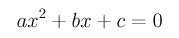A commonly seen formula tells us that the roots are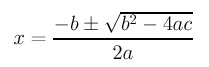If you try a=1, b=-20000 and c=1, the roots computed with single precision are 20000 and 0. This is impossible since the product of the roots is equal to c/a, which is not zero. Where is the problem? If b is very large and both a and c are very small as in the above case, the value of b2-4ac is almost identical to the square of b and as a result, the sum of the square root of this value and -b is zero. While we cannot remove the subtraction in the square root, we can do something to improve this situation. The following offers two such methods:

• Multiplying the roots with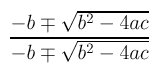would change the roots to the following: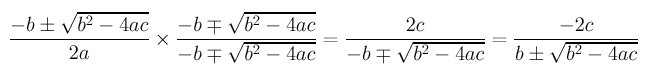If b is positive, the plus sign is chosen. In this case, the denominator is equivalent to adding two positive numbers together and as a result the subtraction is implicitly removed. The first root is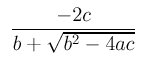If b is negative, we should choose the minus sign and the effect is adding two negative numbers and the subtraction is also removed.

Because the product of the roots is equal to c/a, dividing c/a by the root just found yields the second one. Using this method, the roots are 20000 and 0.00005.

Well, it is still incorrect since the sum of the roots is not equal to 20000. Is it still a problem? Probably not. Since single precision usually provides seven significant digits, the sum of 20000 and 0.00005 is 20000.00005 which will be stored in memory as 0.2000000×105. Therefore, 0.00005 is lost due to normal truncation or rounding. Ah, that is fine.

• In this second method, the given equation is "normalized" first. That is, we change the form of the given equation to the following: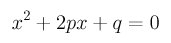where p=b/(2a) and q=c/a. Then, the roots are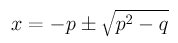Similar to the above method, if p is positive, -p is negative and the minus sign is chosen so that the computation is effectively the sum of two negative numbers. In this case, one of the root is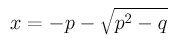On the other hand, if p is negative, -p is positive and the plus sign should be chosen.

After obtaining one of the two roots, the second root is the quotient of dividing q by the the first root since the product of the two roots is q. The computed roots are 20000 and 0.0005, the same as the first method.### Example 2

Let us compute the value of sine using a well-known infinite series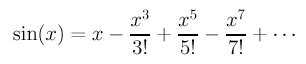Once you see the above series, you perhaps has a feeling that something weird is about to happen, because there are too many subtractions! Yes, it is the case. If we program the sine function as follows:

```float  MySin(float x)
{
float  sinx  = 0.0;
float  term  = x;
float  sign  = 1.0;
float  denom = 1.0;

while (fabs(term) >= EPSILON) {
sinx = sinx + sign*term;
term = term*x/(++denom);
term = term*x/(++denom);
sign = -sign;
}
return  sinx;
}
```
and let the tolerance value for EPSILON be 1.0E-8, we have the following result for x being -30, -25, -20, ..., 20. 25 and 30:
``` x             MySin          sin(x)
---            -----          ------
-30.000000     33002.515625   0.988032
-25.000000     270.567413     0.132352
-20.000000     -0.957176      -0.912945
-15.000000     -0.677538      -0.650288
-10.000000     0.544120       0.544021
-5.000000      0.958925       0.958924
0.000000       0.000000       0.000000
5.000000       -0.958925      -0.958924
10.000000      -0.544120      -0.544021
15.000000      0.677538       0.650288
20.000000      0.957176       0.912945
25.000000      -270.567413    -0.132352
30.000000      -33002.515625  -0.988032
```
the second column is the output of the above program while the third column is computed with the library function sin(). When the value of x is near to zero, the results are almost the same. As the value of x is getting larger, the effect of losing significant digits becomes so severe that the value of the function is not in the range of 0 and 1.

Note that the library function uses some other way to compute the values of sine.

Click here to download a C program of this example. You may want to try adding all positive and negative terms to two variables and using one subtraction to get the result. Compare your result with the bad one above. What do you get?

### Mathematical Laws Sometimes Do Not Work for Finite Precision Computation

This is to warn you that those mathematical laws you are familiar with sometime do not work very well for finite precision computation. Carefully choosing the order of evaluating for your expressions is important. We shall talk about commutative law and associative law only.

The commutative law states that the results of a*b and b*a are the same (in mathematics) for addition and multiplication operators. You have to be careful when you apply this law. Consider computing a*b*c, where a and c are very large numbers and b is very small so that a*b and b*c are close to 1. In this case, computing a*b*c would be fine.

Now let us consider the commutative law by changing the expression to a*c*b. What would happen? You could get an overflow because a*c would be too large to be represented by the hardware!

Associative law does not work well either. The associative law states that one can evaluate a*b*c either by (a*b)*c or by a*(b*c). It looks innocent; but could be damaging.

Consider the following recurrence relations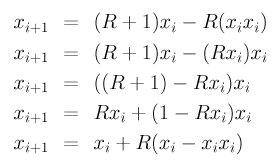where the value for R is 3.0 and the initial value for x is 0.5. These five recurrence relations are equivalent mathematically (you can verify it easily). If we compute the values of x up to the 1000th term and display the results every 100 terms, we have the following shocking results:

```    0           0.5           0.5           0.5           0.5           0.5
100    1.6782e-05       1.30277      0.506627       1.14111      0.355481
200       1.28383       0.97182       1.25677       1.04532      0.805295
300      0.745989      0.155282       1.32845      0.295785     0.0590719
400     0.0744125      0.354702       1.00514      0.882786      0.171617
500      0.788592    0.00176679      0.804004      0.496569       1.32348
600     0.0190271       0.35197      0.832942      0.518201       1.20776
700      0.377182      0.525322       0.31786      0.435079      0.525364
800        0.1293   0.000277146       1.33159     0.0130949      0.954542
900      0.375104    0.00743761       1.27548      0.409476    0.00996984
1000      0.680855       1.03939      0.462035     0.0734742       1.26296
```
All five ways of using the associative law give totally different behavior and this happens very early: the 100th terms are very different!